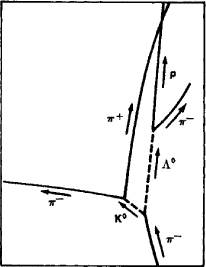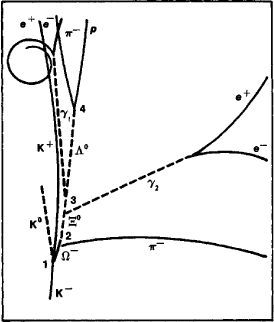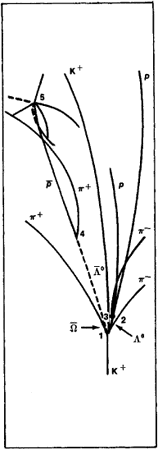# Hyperons

Also found in: Dictionary, Thesaurus.
Related to Hyperons: kaon, sigma baryon
The following article is from The Great Soviet Encyclopedia (1979). It might be outdated or ideologically biased.

## Hyperons

heavy, unstable elementary particles with a mass greater than that of a nucleon (a proton or neutron), which have a baryon charge and a lifetime longer than the “nuclear time” (~10-23 sec). Several types of hyperons are known: lambda (Λ0), sigma (Σ-, Σ0, Σ+), xi (Ξ-, Ξ°), and omega (Ω-). [The superscripts -, 0, and + to the right of the symbols of the particles designate negatively charged, neutral, and positively charged particles, respectively.] All hyperons have a spin of 1/2, except for the Ω-, whose spin should, according to theoretical concepts, be equal to 3/2—that is, hyperons are fermions. Hyperons take part in strong interactions—that is, they belong to the class of hadrons. The lifetime of hyperons is on the order of 10-10 sec (with the exception of 2°, which apparently has a lifetime on the order of 10-20 sec); in this time they decay into nucleons and light particles (π-mesons, electrons, and neutrinos).

Hyperons (Λ0) were discovered in cosmic rays by the English physicists Rochester and Butler in 1947, but convincing proof of their existence was obtained only in 1951. The detailed and systematic study of hyperons became possible when they began to be produced in high-energy charged particle accelerators upon collisions of fast nucleons, π-mesons, and K-mesons with the nucleons of atomic nuclei.

The discovery of hyperons significantly expanded the physical concepts of elementary particles in that particles with a mass greater than nucleonic mass had been discovered for the first time and a new and very important characteristic of elementary particles, strangeness, had been established. The introduction of the concept of strangeness was necessary to explain a number of properties of hyperons that were paradoxical from the standpoint of then-current concepts. The intensive production of hyperons upon collisions of high-energy hadrons indisputably indicated that they have strong interaction. On the other hand, if the decay of hyperons was caused by strong interaction, their lifetime should have been on the order of 10-23 sec, which is 13 orders less than that established experimentally. The lifetime of hyperons can be explained if it is assumed that their decay occurs through weak interaction, whose relative intensity in this energy region is indeed 12-14 orders less than that of the strong interaction (and hence the decay time is correspondingly longer). It seemed a paradox that particles that have strong interaction could not decay by means of that interaction.

The fact that, upon collision of π-mesons and nucleons with nucleons, hyperons are always produced, togetherFigure 1. Schematic diagram of a case of pair production of a Λ0-hypheron and a K0-meson in a proton in a liquid-hydrogen bubble chamber under the influence of a π- meson: π- + p → Λ0 + K0. This reaction resulted from strong interaction and is permitted by the law of conservation of strangeness (the total strangeness of particles in the initial and final states is identical and equal to zero). The decays of a Λ0-hyperon and a K0-meson under the force of weak interaction are also visible: Λ0 → p + π-, K0 → π+ + π- (in each of these processes the strangeness changes by 1). The dotted lines show the paths of neutral particles, which leave no tracks in the chamber.

with K-mesons (Figure 1), in whose behavior the same strangenesses are detected as with hyperons, was of great importance in solving this paradox. The peculiarities of the behavior of hyperons and K-mesons were explained in 1955 by Gell-Mann and Nishijima in terms of the existence of a special characteristic of hadrons—strangeness (S), which is conserved in the processes of strong and electromagnetic interaction. If K+- and K°-mesons are assigned a strangeness S = +1 and A-hyperons and Λ-hyperons are assigned a strangeness equal in magnitude but opposite in sign, S = -1, and if the strangeness of π-mesons and nucleons is assumed to be equal to zero, then the conservation of the total strangeness of the particles in strong interactions also explains both the joint production of Λ- and Σ-hyperons with K-mesons and the impossibility of the decay of particles havingFigure 2. Schematic diagram of a case of production and decay of an Ω--hyperon in a liquid-hydrogen bubble chamber. The Ω- hyperon is produced (at point 1) when a K- meson collides with a photon in the reaction K- + p → Ω- + K+ + K0, which is due to strong interaction and is permitted by the law of conservation of strangeness S (in the initial and final states S = -1). The decays of the particles formed take place as a result of weak interaction, and the strangeness changes by 1: Ω- → Ξ0 + π0 (at point 3), and π0, which has a short lifetime, decays virtually at the same point 3 into two γ-quanta, π0 → γ1 + γ2 which produce electron-positron pairs e+, e-; Λ0 → p + π- (at point 4). The tracks of the particles are curved, since the chamber is in a magnetic field.

a strangeness other than zero (such particles have been given the name “strange particles”) by means of strong interactions on particles of zero strangeness. Here Ξ-hyperons, which are produced together with two K-mesons, should be given S = -2, and Ω--hyperons S = -3. Decays of hyperons indicate that the processes caused by weak interactions transpire with a change of strangeness. The processes of the strong and weak interaction of hyperons are illustrated in Figure 2.

According to the modern theory of elementary particles, an antiparticle that differs from its own hyperon in the sign of electrical and baryon charges and of strangeness should correspond to each hyperon. All antihyperons havebeen experimentally observed: the antiomega hyperon, Ω, or Ω+ (Figure 3), was the last to be discovered (in 1971).Figure 3. Schematic diagram of a case of production and decay of the antihyperon Ω (Ω+) in a bubble chamber filled with liquid deuterium and located in a magnetic field. The antihyperon Ω, which has positive electrical charge and a strangeness of S = +3, is produced (at point 1) when a K+-meson (with an energy of 12 giga electron volts) collides with a deuterium nucleus in the reaction K+ + d → Ω + Λ0 + Λ0 + p + π+ + π-. According to the laws of the conservation of baryon charge î and (in a strong interaction) strangeness S, the production of the antibaryon Ω (B = -1) in a deutron (B = +2) is accompanied by the production of three baryons: Λ0, Λ0, and p (the strangeness of the system in the initial state is determined by the strangeness of K+ and is equal to S = +1). The decays of the particles formed take place as a result of weak interaction, and the strangeness changes by 1. One of the Λ0 that forms decays (at point 2) into p and π-, and the other leaves the chamber before it can decay (but its presence is confirmed by the law of conservation of energy and momentum); the antihyperon Ω decays (at point 3) into the anti-lambda hyperon Λ° and K+; Ξ0 decays (at point 4) into an anti-proton p and π+ and is annihilated (at point 5) with a proton, forming several π-mesons.

Strong interaction In addition to the conservation of strangeness, strong interactions of hyperons have a certain symmetry

was previously established for nucleons and π-mesons and is manifested in the fact that particles are grouped into certain families—isotopic multiplets [(p, n) and (π-, π0, π+), where p designates a proton and n a neutron]. Particles that are part of a certain isotopic multiplet participate identically in strong interaction, have nearly equal masses, and differ only in their electromagnetic characteristics (electrical charges and magnetic moments). The number of particles in an isotopic multiplet is characterized by a special quantum number—the isotopic spin I —and is equal to 2I + 1. Hyperons form four isotopic multiplets (see Table 1).

The assumption of the existence of isotopic multiplets of hyperons enabled Gell-Mann and Nishijima to predict the existence of Σ0 and Ξ0 before their experimental discovery.

In a number of properties the Λ-, Σ-, and Ξ-hyperons are analogous to nucleons. This analogy served as the starting point in the search for the symmetry of strong interactions, which is broader than isotopic invariance. So-called unitary symmetry (SU3-symmetry), from which the hadron system was developed, has produced the greatest success in this process. By using this symmetry it has proved possible, for example, to predict the existence and properties of the Ω--hyperon.

Hyperon decay The main types of decay of hyperons are indicated in Table 1. Hyperon decays conform to the following rules:

(1) ΔS = 1—the strangeness changes by one unit in absolute magnitude; an exception is the decay of Σ0 into Λ0 and a photon, Σ0→ Λ + γ, which takes place through electromagnetic interaction (hence the lifetime of Σ0 should be ~10-20, not 10-10 sec) and which therefore is not accompanied by a change in strangeness. This law prohibits the direct decay of a Ξ-hyperon into a nucleón and π-mesons, since upon such decay the strangeness would change by two units. The decay of a H-hyperon takes place in two stages: Ξ→Λ0 + π; Λ0→N + π(where N designates a nucleon). Therefore the Ξ-hyperon is called a cascade hyperon; Ω--hyperons also undergo cascade decays.

(2) ΔQ = ΔS —in decays involving the emission of leptons the change in the charge Q of the hadrons is equal to the change in the strangeness S. This law forbids, for example, the decay Σ+→n + μ+ + v++ is a positive muon, v is a neutrino).

(3) ΔI = 1/2—isotopic spin changes by 1/2. This rule makes it possible to explain the relations among the probabilities of the various observed modes of decay of hyperons.

Hypernuclei, in which one or several nucleons are converted into hyperons as a result of strong interaction, may be formed upon interaction between fast particles and nuclei.

### REFERENCES

Gell-Mann, M., and P. E. Rosenbaum. “Elementarnye chastitsy.” In Elementarnye chastitsy. Moscow, 1963. (Translated from English; Nad chem dumaiut fiziki, issue 2.)
Adair, R. K., and E. K. Fowler. Strannye chastitsy. Moscow, 1966. (Translated from English.)
Frisch, D., and A. Thorndike. Elementarnye chastitsy. Moscow, 1966. (Translated from English.)

L. G. LANDSBERG

References in periodicals archive ?
Most physicists stretch the term "ordinary matter" to include bizarre particles like hyperons that exist only inside atom smashers.
For kaons and hyperons, however, the strangeness number was not 0 but +1, -1, +2, or -2.
Notice that similar interaction Hamiltonian, but with other coupling constants, describes the neutrino weak interaction of hyperons in NS matter (e.g., ).
This makes the neutrino radiation from [sup.1][S.sub.0] pairing of protons or hyperons unimportant.
This makes the PBF neutrino losses from pairing of protons or hyperons unimportant.
Prakash, "Kaon condensation in neutron star matter with hyperons," Physics Letters B, vol.
Gmuca, "Hyperons in a relativistic mean-field approach to asymmetric nuclear matter," Physical Review C Nuclear Physics, vol.
Teryaev, "Femto-vortex sheets and hyperon polarization in heavy-ion collisions," Physical Review C, vol.
Upsal, "Observation of global hyperon polarization in ultrarelativistic heavy ion collisions," Journal of Physics: Conference Series, vol.
Corrected fractions of observed, [K.sup.0.sub.s] and [LAMBDA] decays that originate from the decays of strange resonances and heavy hyperons were found to be (15.5 [+ or -] 0.9)%, (8.7 [+ or -] 0.7)%, (5.8 [+ or -] 1.1)%, (2.6 [+ or -] 0.8)%, (7.3 [+ or -] 2.4)%, and (1.9 [+ or -] 1.7)% for the channels [K.sup.*+] [right arrow] [K.sup.0.sub.s][[pi].sup.+], [K.sup.*-] [right arrow] [K.sup.0.sub.s][[pi].sup.-], [[SIGMA].sup.*+][right arrow][LAMBDA][[pi].sup.+], [[SIGMA].sup.*-] [right arrow] [LAMBDA][[pi].sup.-], [[SIGMA].sup.0] [right arrow] [LAMBDA][gamma], and [[XI].sup.-] [right arrow] [LAMBDA][[pi].sup.-], respectively.
The study of A polarization relied on an efficient and robust [LAMBDA] hyperon identification algorithm and a high statistics sample.
Popov, "Measurement of the A hyperon polarization in [v.sub.[mu]] charged current interactions in the NOMAD experiment," Nuclear Physics A, vol.

Site: Follow: Share:
Open / Close